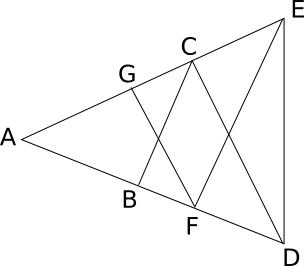# CAT 2000

Instructions

For the following questions answer them individually

Question 151

# If a,b,c are the sides of a triangle, and $$a^2 + b^2 +c^2 = bc + ca + ab$$, then the triangle is:Question 152

# In the figure AB=BC=CD=DE=EF=FG=GA, then $$\angle{DAE}$$ is approximatelyQuestion 153

# A shipping clerk has five boxes of different but unknown weights each weighing less than 100 kgs. The clerk weighs the boxes in pairs. The weights obtained are 110, 112, 113, 114, 115, 116, 117, 118, 120 and 121 kgs. What is the weight, in kgs, of the heaviest box?Question 154

# There are three cities A, B and C. Each of these cities is connected with the other two cities by at least one direct road. If a traveller wants to go from one city (origin) to another city (destination), she can do so either by traversing a road connecting the two cities directly, or by traversing two roads, the, first connecting the origin to the third city and the second connecting the third city to the destination. In all there are 33 routes from A to B (including those via C). Similarly there are 23 routes from B to C (including those via A). How many roads are there from A to C directly?Question 155

# The set of all positive integers is the union of two disjoint subsets:{f(1), f(2),.....f(n), ...} and {g(1),g(2).... ,g(n).....}, where f(1) < f(2) <.....< f(n)..., and g(1) < g(2) < ..... < g(n) ...,andg(n) = f(f(n))+1 for all n >= 1. What is the value of g(1)?Question 156

# ABCDEFGH is a regular octagon. A and E are opposite vertices of the octagon. A frog starts jumping from vertex to vertex, beginning from A. From any vertex of the octagon except E, it may jump to either of the two adjacent vertices. When it reaches E, the frog stops and says there. Let $$a_n$$ be the number of distinct paths of exactly n jumps ending in E. Then, what is the value of $$a_{2n-1}$$?Question 157

# For all non-negative integers x and y, f(x, y) is defined as below:f(0, y) = y + 1f(x + 1, 0) = f(x, 1)f(x+ 1, y+ 1)= f(x, f(x+ 1, y))Then, what is the value of f(1,2)?Question 158

# Convert the number 1982 from base 10 to base 12. The result is:Question 159

# Two full tanks, one shaped like a cylinder and the other like a cone, contain jet fuel. The cylindrical tank holds 500 litres more than the conical tank. After 200 litres of fuel has been pumped out from each tank the cylindrical tank contains twice the amount of fuel in the conical tank. How many litres of fuel did the cylindrical tank have when it was full?Question 160

# A farmer has decided to build a wire fence along one straight side of this property. For this, he planned to place several fence-posts at six metre intervals, with posts fixed at both ends of the side. After he bought the posts and wire, he found that the number of posts he had bought was five less than required. However, he discovered that the number of posts he had bought would be just sufficient if he spaced them eight metres apart. What is the length of the side of his property and how many posts did he buy?OR